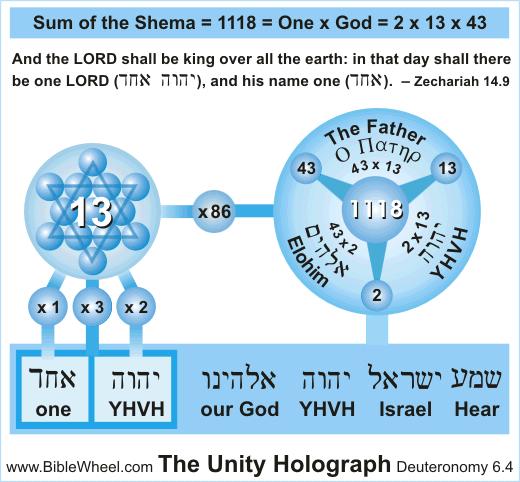# meaning of number 1 — beginnings, unity, Alpha

Deuteronomy 6:4
“Hear, O Israel: The Lord our God is one Lord:”

The first meaning of number 1 is that it stands for beginnings. The first verse of the Bible starts with “In the beginning”, and the first verse of John 1:1 is the only verse that starts with “In the beginning”.
Verse number 1 of the Bible is Genesis 1:1 with a total value of 2701. Verse number 2701 has a total value of = 1981 = total value of Song of Solomon 1:1!
These are great indicators that the first meaning of number 1 concerns beginnings.
-<>-
-<>-
Richard Amiel McGough (Biblewheel.com) discovered a great relationship between number 1 in “the Lord”, “God”, & “the Father”.
Number 1 is also seen in the Shema (Deu 6:4). And this gives us a clear picture that the secondary meaning of number 1 is unity.
The Shema displays a clear connection to number 1 and God. The Hebrew spelling of “one” אחד has a value of 13.
Number 13 is a prime factor of 26 “the Lord” יהוה. The value of “God” אלהים equals 86 (2×43).
And the Greek spelling of “The Father” Ο Πατηρ = 13×43 (factors in “The Lord” & “God”).
-<>--<>-
-<>-

-<>-
meaning of number 1 and α the fine-structure constant

-<>-

The third meaning of number 1 is the fact that it is connected to the fine-structure constant (α).
Number 1 is the value of the first Greek-letter Alpha α. And the most important & mysterious number in physics is the fine-structure constant, which is represented by the first Greek letter Alpha α.
-<>-
the fine-structure constant is: (inverse α) 1/137… = 0.00729… (α)
And we see that the ultra calculation of standard Vs(1)/ultra calculation of reverse standard Vs(137) = 0.0000002701… – – – 2701 = total value of Vs(1 “α”).
An ultra calculation is a nickname for the number of letters x product of letters divided by number of words x product of words.
-<>-
Alpha the fine-structure constant in Genesis 1:1
Word number 137 by rotation when counting in cycles by the number of words in Vs(1 “α”) is the center word.

• The value of the center word = 401 = 400 + 1 “α” = Pythagorean prime number 38 “Alpha” (Eng ord).
• The center word is the only word that is a prime (401 = Pri(80)). And adding center words of verses ordered at 1 & 401 = 769 = Pri(137 (inverse α)).
729 (α) = 100x(3 = Lucky(7)) + reduced, ordinal & standard values added of the center word.
• There are three triangular numbers found in Genesis 1:1. Now divide from the largest to the lowest: 2701/703/28 = 0.137 (inverse α).

• First & last words added divided by 3 center words is 1209/882 = 1370… (4 digits of inverse α).
• First 3 center words divided by first & last words added is 882/1209 = 729… (3 digits of α).
• The verse order added with the number of words & letters joined into one number is 1+728 = 729 (α).
• Total value of verse number 1 = 2701. And the total value of verse number 2701 = 1981 = the total value of the first verse of Song of Solomon.
Alpha is extremely connected to number 777 and the Bible is full of references to this. therefore we see the reverse standard value of the first verse of Song of Solomon = 777

-<>-
And on and on it goes. Fun fact: The codes of Alpha (and Phi) are so fundamental that it is seen in every single chapter of my book.
But as you may see, number 1 has everything to do with Alpha.
-<>-
So, the third and most secret meaning of number 1 is that it stands for Alpha the fine-structure constant.

1 = α [H3739 – – – 37 = prime factor of Genesis 1:1 – – – 39 = factor of John 1:1]
First found as a single word in Vs(23161 = (E r) “codes” 19 x TV of Vs(86 “of God” אלהים) = היקום “the universe” 161 x 1000×23 “riddles” חידות (r))

Number 1 is the first prime number in the eyes of God (and number 2 is the first prime in the eyes of man).
Number 1 is the first polygonal number of all polygons except rectangular numbers.
913 “In the beginning” בראשית = Happy(130 = 10x 13 “one” אחד)

error: Alert: Content is protected !!